Home | | Finite Element Analysis | One Dimensional Finite Element Analysis: Beam Element

# One Dimensional Finite Element Analysis: Beam Element

A beam element is defin ed as a long, slender member (one dimension i s much larger than the other two) that is subje cted to vertical loads and moments, which pro duce vertical displacements and rotations.

BEAM ELEMENT

A beam element is defin ed as a long, slender member (one dimension i s much larger than the other two) that is subje cted to vertical loads and moments, which pro duce vertical displacements and rotations. T he degrees of freedom for a beam element are a vertical displacement and a rotation at e ach node, as opposed to only an horizontal di splacement at each node for a truss element.

Degrees of Freedom

Degrees of freedom are defined as the number of independent coordina tes necessary to specify the configuration of a system. The degrees of freedom for a general situation consists of three translations in t he x, y, and z directions and three rotations abou t the x, y, and axes. A one-dimensional beam element has four degrees of freedom, which include, a vertical displacement and a rot ation at each node.Assumptions

Nodal Forces and Moments

Forces and moments can only be applied at the nodes of the beam element, not between the nodes. The nodal forces and moments, fc, are related to the nodal displacements and rotations,through the ele ment stiffness matrix,Kv.

The loads that are appli ed to the beam element are assumed to be stat ic and not to vary over the time period being considered, this assumption is only valid if the r ate of change of the force is much less than th e applied force (F >> dF/dt). If the loads vary significantly, (if the variation in load is not much less than the applied force) then the pro blem must be considered as dynamic.

Weightless Member

The weight (W) of the beam is neglected, if it is much less than the total resultant forces (F) acting on the beam. If the weight of the beam is not neglected, then its effects must be represented as vertical forces acting at the nodes, by dividing up the weight and lumping it at the nodes, proportionally according to it's placement along the beam.

Prismatic Member

The beam element is assumed to have a constant cross-section, which m eans that the cross-sectional area and the mom ent of inertia will both be constant (i.e., the be am element is a prismatic member). If a bea m is stepped, then it must be divided up into sections of constant cross-section, in order to obtain an exact solution. If a beam is tape red, then the beam can be approximated by us ing many small beam elements, each having the same cross-section as the middle of the tap ered length it is approximating. The more sections that are used to approximate a tapered be am, the more accurate the solution will be.

The moment of inertia is a geometric property of a beam element, which describes the beams resistance to bending and is assumed to be constant through the length of the element. The moment of inertia can be different along different axes if the beam el ement is not symmetric, we use the moment of inertia (I) of the axis about which the bendin g of the beam occursWhere (Iz) refers to the moment of inertia, resisting bending about the "z" axis and (Iy) about the "y" axis.

The Beam Element is a Slende r Member

A beam is assumed to be a slender member, when it's length (L) is moree than 5 times as long as either of it's cross-sec tional dimensions (d) resulting in (d/L<.2). A beam must be slender, in order for the beam equations to apply, that were used to derive our FEM equations.The Beam Bends without Twisting.

It is assumed that the c ross-section of the beam is symmetric about the plane of bending (x-y plane in this case) and will undergo symmetric bending (where n o twisting of the beam occurs during the bend ing process). If the beam is not symmetric abo ut this plane, then the beam will twist during bending and the situation will no longer be on e-dimensional and must be approached as an u nsymmetric bending problem (where the beam twists while bending) in order to obtain a cor rect solution.

Cross Section Remains Plane

When a beam element b ends, it is assumed that it will deflect uniformly, thus the cross section will move uniform ly and remain plane to the beam centerline. In other words, plane sections remain plane and normal to the x axis before and after bending.

Axially Rigid

The one-dimensional bea m element is assumed to be axially rigid, mean ing that there will be no axial displacement ( u) along the beams centriodal axis. This implies that forces will only be applied perpendicular to the beams centriodal axis. The one-dimensional beam element can be used only when the degrees of freedom are limited to vertical displacements (perpendicular to the beams centriodal axis) and rotations in one plane. If axial displacements are present then a one-dimen sional bar element must be superimposed w ith the one-dimensional beam element in ordder to obtain a valid solution.

Homogenous Material

A beam element has the s ame material composition throughout and there fore the same mechanical properties at every position in the material. Therefore, the modulus of elasticity E is constant throughout the beam element. A member in which the material properties varies from one point to the next in t he member is called inhomogenous (non-homo genous). If a beam is composed of different ty pes of materials, then it must be divide up into elements that are each of a single homogeneous material, otherwise the solution will not be exact.

Isotropic Material

A beam element has the same mechanical and physical properties in all directions, i.e., they are independent of dir ection. For instance, cutting out three tensile test specimens, one in the x-direction, one in t he y-direction and the other oriented 45 degre es in the x-y plane, a tension test on each s pecimen, will result in the same value for th e modulus of elasticity (E), yield strength  and ultimate strength. Most metals arre considered isotropic. In contrast fibrous m aterials, such as wood, typically have properties that are directionaly dependant and are generally considered anisotropic (not isotropic).

The Proportional Limit is not Exceeded

It is assumed that the b eam element is initially straight and unstressed. It is also assumed that the material does n ot yield, therefore the beam will be straight af ter the load is released. These assumptions me an that the beam must be made of an elastic material, one which will return to it's original size and shape when all loads are removed, if not stressed past the materials elastic or proportional limit. It is also assumed that the beam is not stressed past the proportional limit, at w hich point the beam will take a permanent set and will not fully return to it's original size an d shape, when all loads are removed. Below th e proportional limit an elastic material is in the linear elastic range, where the strain  varies linearly with the applied load and the stress (  ) varies linearly according to: σ = Eε  , where E is the     modulus of elasticity.

Rigid Body Modes for the One-Dimensional Beam Element

Rigid Body Modes for the One-Dimensional Beam Element

Rigid body motion occurs when forces and/or moments are applied to an unrestrained mesh (body), resulting in motion that occurs without any deformations in th e entire mesh (body). Since no strains (deformations) occur during rigid body motion, there can be no stresses developed in the mesh. In order to obtain a unique FEM solution, rigid body motion must be constrained. If rigid body motion is not constrained, then a singular system of equations will result, since the determinate of the mesh stiffness matrix is equal to zero (i.e., ( |K| - 0)

There are two rigid body modes for the one-dimensional beam element , a translation (displacement) only and a rotation only. These two rigid body modes can occu r at the same time resulting in a displacement and a rotation simultaneously. In order to e liminate rigid body motion in a 1-D beam elem ent (body), one must prescribe at least two no dal degrees of freedom (DOF), either two displacements or a displacement and a rotation. A DOF can be equal to zero or a non-zero know n value, as long as the element is restrained fro m rigid body motion (deformation can take pl ace when forces and moments are applied) .

For simplicity we will in troduce the rigid body modes using a mesh c omposed of a single element. If only translatio nal rigid body motion occurs, then the displaceement at local node I will be equal to the displacement at local node J. Since the displacem ents are equal there is no strain developed in t he element and the applied nodal forces cause the element to move in a rigid (non-deflected) vertical motion (which can be either up as show n below or it can be in the downward directio n depending on the direction of the applied force s).This rigid body mode can be suppressed by prescribing a vertical nodal displace ment.

If rotational rigid body motion occurs, then the rotation at local node I will be equal to the rotation at local node J (i.e., in magnitude and direction). In this situation th e nodal forces and/or moments applied to the element, cause the element to rotate as a rigid body (either clockwise as shown below or counterclockwise depending on the direction o f the applied forces and/or moments).This rigid body mode can be suppressed by prescribing a nodal translation or rotation.

If translational and rotational rigid body motion occurs simultaneously then:1-D 2-NODED CUBIC BE AM ELEMENT MATRICES

A single 1-d 2-noded c ubic beam element has two nodes, with two degrees of freedom at each node (one vertic al displacement and one rotation or slope). The re is a total of 4 dof and the displacement polynomial function assumed should have 4 terms, so we choose a cubic polynomial for the vertical deflection. Slope is a derivative of the vertical deflections.DEVELOPMENT OF ELEMENT EQUATION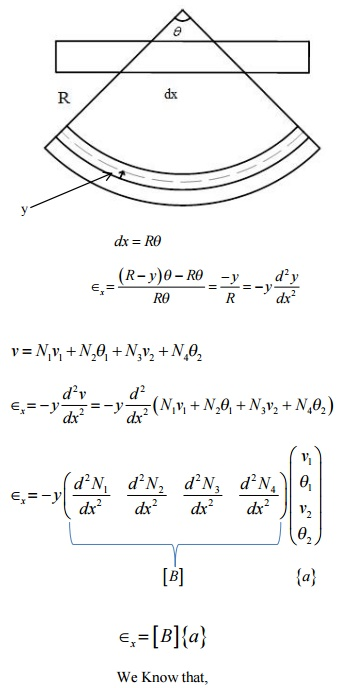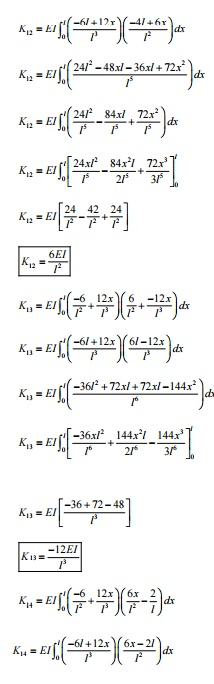BEAM ELEMENT

A beam is a long, slender structural member generally subjected to transverse loading that produces significant bending effects as opposed to twisting or axial effects. An elemental length of a long beam subjected to arbitrary loading is considered for analysis. For this elemental beam length L, we assign two points of interest, i.e., the ends of the beam, which become the nodes of the beam element. The bending deformation is measured as a transverse (vertical) displacement and a rotation (slope). Hence, for each node, we have a vertical displacement and a rotation (slope)  two degrees of freedom at each node. For a single 2-noded beam element, we have a total of 4 degrees of freedom. The associated “forces” are shear force and bending moment at each node.The stiffness term kij indicates the force (or moment) required at i to produce a unit deflection (or rotation) at j, while all other degrees of freedom are kept zero.

Sign conventions followed

Upward forces are positive and upward displacements are positive.

Counter-clockwise moments are positive and counter-clockwise rotations are positive.

Formulae required  cantilever beam subjected to concentrated load and moment.1 ELEMENT MATRICES AND VECTORS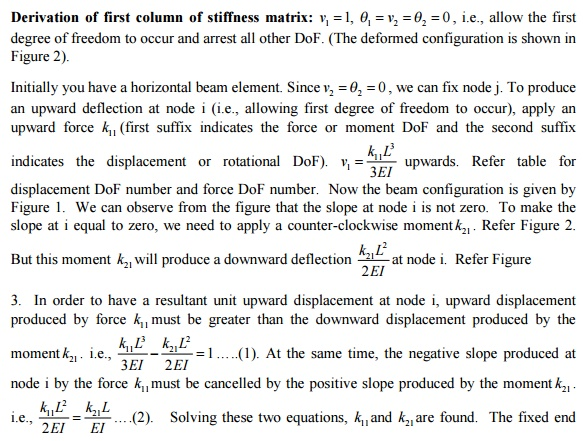reaction force and the reaction moment are assumed to be acting upwards and counterclockwise, respectively. Now use force equilibrium equation to find fixed end reaction force k  ….…Derivation of second column of stiffness matrix:found. The fixed end reaction force and the reaction moment are assumed to be acting upwards and counterclockwise, respectively. Now use force equilibrium equation to find fixed  end          reaction forceDerivation of third column of stiffness matrix:

Derivation of third column of stiffness matrix:  v1        = 0, q1 =0, v2    1,= q      2        0 , i.e., allow the third degree of freedom to occur and arrest all other DoF. (The deformed configuration is shown in Figure 2).

Initially you have a horizontal beam element. Since V1=q1=0 we can fix node i. To produce an upward deflection at node j (i.e., allowing third degree of freedom to occur), apply an upward force k33 .upwards. Now the beam configuration is given by Figure 1. We can observe from 3EIthe figure that the slope at node j is not zero. To make the slope at j equal to zero, we need to apply a clockwise moment k43 . Refer Figure 2. But this moment k43 will produce a downward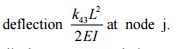at node j. Refer Figure 3. In order to have a resultant unit upward 2EI displacement at node j, upward displacement produced by force k33 must be greater than thesame time, the positive slope produced at node j by the force k33 must be cancelled by the negative slope produced by the moment k43Solving these two equations, k33 and k43 are found. The fixed end reaction force and the reaction moment are assumed to be acting upwards and counterclockwise, respectively. Now use force equilibrium equation to find fixed end reaction forceand moment equilibrium equation about node i   to  find fixed end reaction moment   k23   ....Derivation of fourth column of stiffness matrix:fourth degree of freedom to occur and arrest all other DoF. shown in Figure 2).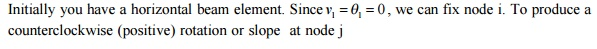i.e., allow the (The deformed configuration is shown in fig.

Initially you have a horizontal beam element.

Since, we can fix node i. To produce a counterclockwise (positive) rotation or slope  at node j (i.e., allowing fourth degree of freedom  to occur), apply a counterclockwise moment k44 .  Refer Figure 1. This moment k44 will produce a upward deflectionThis upward deflection should be canceled by applying aRefer Figure 3. Solving these two equations, k34 and k44 are found. The fixed end reaction force and the reaction moment are assumed to be acting upwards and counterclockwise, respectively.

Now  use force  equilibrium equation to find   fixed   end   reaction   force   k14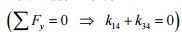and moment equilibrium equation about node i to find fixed end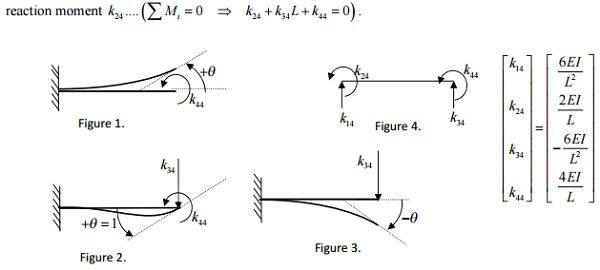Problem

Find the slopes at the supports and support reac beam shown in Figure-.4m4. TakeDarylP4E=210-24LoganpageGPa,208I. = 2×10Finite element representation of the problem

Conversion of UDL into nodal forces and nodal moPROBLEM

Given that E=210 GPa and I=4×10 -4 m4, cross section of the beam is constant. Determine the deflection and slope at point C. calculate the reaction forces and moments. DARYL LOGAN P 171-172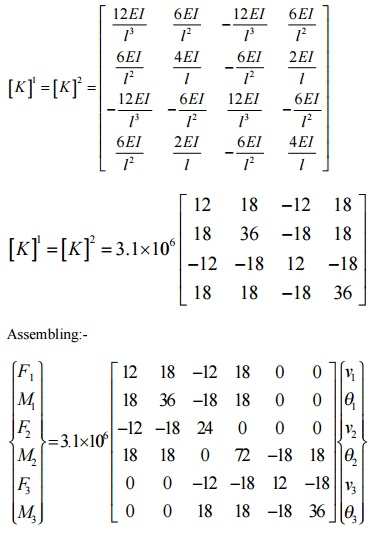Boundary condition:-

F2=-10 kN; M2=20 kN-m

Therefore first, second, fifth, sixth columns are ineffective and hence the reduced matrix is given byDeflection and slope at point c:-

V2= -1.34×10 -4 m = -0.134 mm

Reaction forces and moments:-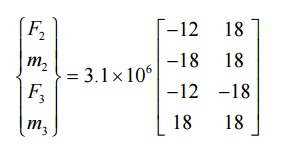F1=10000N

M1=12500N-m

F3=0

M3= -2500N-m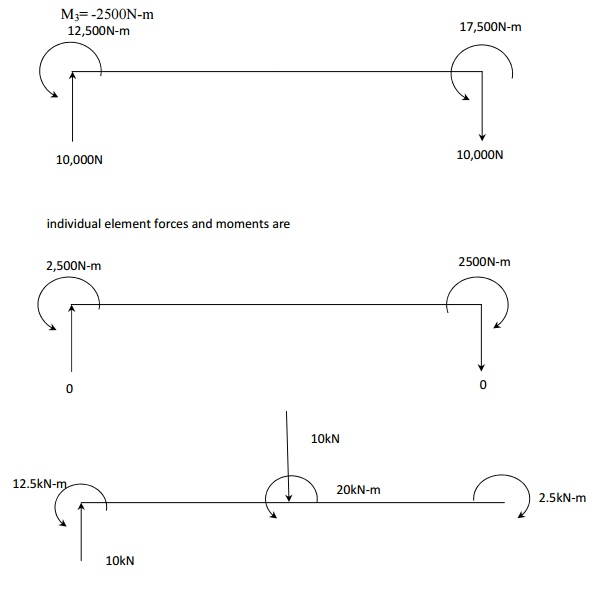Study Material, Lecturing Notes, Assignment, Reference, Wiki description explanation, brief detail
Mechanical : Finite Element Analysis : One Dimensional Finite Element Analysis : One Dimensional Finite Element Analysis: Beam Element |